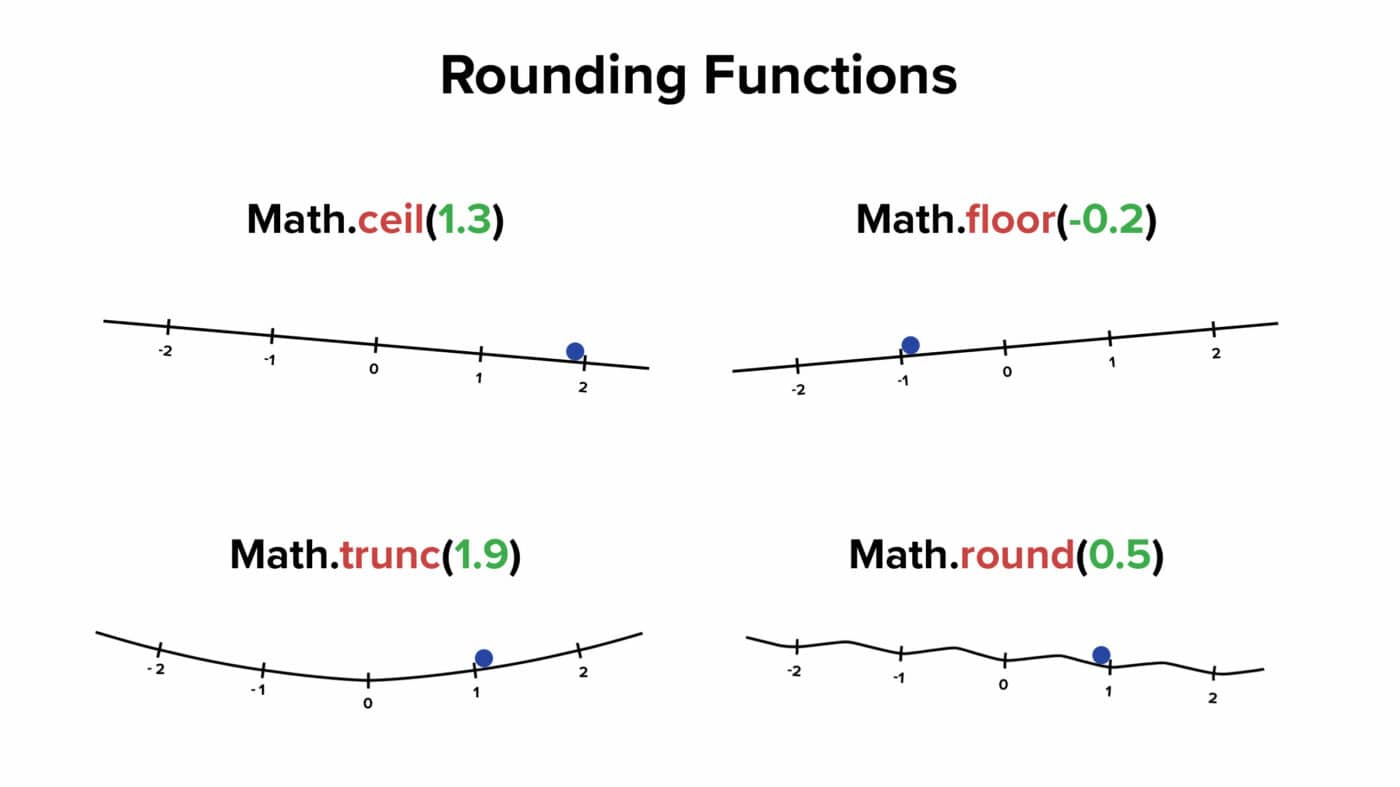# ceil

Learn how to use the ceil function in Notion formulas.

The `ceil()` function returns the smallest integer that is greater than or equal to its argument.

```.wp-block-code {
border: 0;
}

.wp-block-code > div {
overflow: auto;
}

.shcb-language {
border: 0;
clip: rect(1px, 1px, 1px, 1px);
-webkit-clip-path: inset(50%);
clip-path: inset(50%);
height: 1px;
margin: -1px;
overflow: hidden;
position: absolute;
width: 1px;
word-wrap: normal;
word-break: normal;
}

.hljs {
box-sizing: border-box;
}

.hljs.shcb-code-table {
display: table;
width: 100%;
}

.hljs.shcb-code-table > .shcb-loc {
color: inherit;
display: table-row;
width: 100%;
}

.hljs.shcb-code-table .shcb-loc > span {
display: table-cell;
}

.wp-block-code code.hljs:not(.shcb-wrap-lines) {
white-space: pre;
}

.wp-block-code code.hljs.shcb-wrap-lines {
white-space: pre-wrap;
}

.hljs.shcb-line-numbers {
border-spacing: 0;
counter-reset: line;
}

.hljs.shcb-line-numbers > .shcb-loc {
counter-increment: line;
}

.hljs.shcb-line-numbers .shcb-loc > span {
}

.hljs.shcb-line-numbers .shcb-loc::before {
border-right: 1px solid #ddd;
content: counter(line);
display: table-cell;
text-align: right;
-webkit-user-select: none;
-moz-user-select: none;
-ms-user-select: none;
user-select: none;
white-space: nowrap;
width: 1%;
}```ceil(number)
``````

In other words, `ceil()` rounds a non-whole number to the next largest integer. When used on an integer, it will return that integer.

``````ceil(4.2) // Output: 5

ceil(3.845) // Output: 4

ceil(4) // Output: 4

// Calculate the donated change in a round-up donation
// Assume prop("Subtotal") is \$5.34
ceil(prop("Subtotal")) - prop("Subtotal")
// Output: \$0.66
``````

It’s worth noting that `ceil()` rounds “upward towards positive infinity”, while its counterpart, floor, rounds “downward towards negative infinity”Note: This graphic shows JavaScript rounding functions. Notion formulas don’t have a trunc() function, but you can create one using ternary syntax (shown below).

When a negative value is passed as `ceil()`‘s argument, you’ll still get a greater value.

``````ceil(-3.7) // Output: -3
``````

`ceil()` does not round “away from zero”, and floor does not round “towards” zero.

In JavaScript, the Math.trunc function rounds “towards” zero. It simply removes all decimal values from a non-whole number – e.g. `Math.trunc(3.7) == 3` and `Math.trunc(-3.7) == -3`.

Notion does not have a `trunc()` function, but you can create one using an if-then statement:

``````// Assume a number property exists called Num
prop("Num") >= 0 ? floor(prop("Num")) : ceil(prop("Num"))
``````

You can see a working example database showing this formula in action in the floor article.

Stores often ask customers if they would like to make a donation to charity along with their purchase.

This example database calculates donations based on four possible options – \$1, \$5, Round-Up, and no donation.

A “round-up” donation simply rounds the purchase sub-total to the next greatest whole dollar, and donates the change.

``````// Compressed
if(contains(prop("Donation Choice"),"\$"),toNumber(replaceAll(prop("Donation Choice"),"[^\\d]","")),if(prop("Donation Choice") == "Round-Up",ceil(prop("Subtotal")) - prop("Subtotal"),0))

// Expanded
if(
contains(
prop("Donation Choice"),
"\$"
),
toNumber(
replaceAll(
prop("Donation Choice"),
"[^\\d]",
""
)
),
if(
prop("Donation Choice") == "Round-Up",
ceil(prop("Subtotal")) - prop("Subtotal"),
0
)
)
``````

The Donation Choice select property models multiple-choice buttons that customers may press on a point-of-sale system.

This formula calculates the donation based on the customer’s choice of \$1, \$5, Round-Up, or no donation.

A nested if statement is used to check which option was chosen, and to execute different logic depending on the choice.

First, the contains function is used to check if the choice contains `\$`. If so, we know that the choice was either \$1 or \$5.

Since Select options are strings by default, we use replaceAll and a small regular expression to remove any non-numeric character from that string (`[^\\d]` translates to “any character that isn’t a digit”).

Then we use toNumber to convert the resulting string to an actual number and output it.

If the Select option doesn’t contain a `\$`, then we check if it is equal to `Round-Up`.

If so, we use `ceil()` to find the amount of change donated in the round-up donation: `ceil(prop("Subtotal")) - prop("Subtotal")`.

Finally, if the `None` option was chosen, we set the donation to `\$0`.